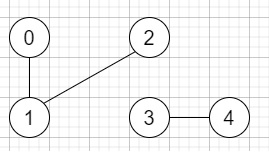# Number of Connected Components in an Undirected Graph in C++

Suppose we have n nodes and they are labeled from 0 to n - 1 and a list of undirected edges, are also given, we have to define one function to find the number of connected components in an undirected graph.

So, if the input is like n = 5 and edges = [[0, 1], [1, 2], [3, 4]],then the output will be 2

To solve this, we will follow these steps −

• Define a function dfs(), this will take node, graph, an array called visited,

• if visited[node] is false, then −

• visited[node] := true

• for initialize i := 0, when i < size of graph[node], update (increase i by 1), do −

• dfs(graph[node, i], graph, visited)

• From the main method do the following −

• Define an array visited of size n

• if not n is non-zero, then −

• Define an array graph[n]

• for initialize i := 0, when i < size of edges, update (increase i by 1), do −

• u := edges[i, 0]

• v := edges[i, 1]

• insert v at the end of graph[u]

• insert u at the end of graph[v]

• ret := 0

• for initialize i := 0, when i < n, update (increase i by 1), do −

• if not visited[i] is non-zero, then −

• dfs(i, graph, visited)

• (increase ret by 1)

• return ret

## Example

Let us see the following implementation to get better understanding −

Live Demo

#include <bits/stdc++.h>
using namespace std;
class Solution {
public:
void dfs(int node, vector<int< graph[], vector<bool>& visited){
if(visited[node]) return;
visited[node] = true;
for(int i = 0; i < graph[node].size(); i++){
dfs(graph[node][i], graph, visited);
}
}
int countComponents(int n, vector<vector<int<>& edges) {
vector <bool> visited(n);
if(!n) return 0;
vector <int< graph[n];
for(int i = 0; i < edges.size(); i++){
int u = edges[i];
int v = edges[i];
graph[u].push_back(v);
graph[v].push_back(u);
}
int ret = 0;
for(int i = 0; i < n; i++){
if(!visited[i]){
dfs(i, graph, visited);
ret++;
}
}
return ret;
}
};
main(){
Solution ob;
vector<vector<int<> v = {{0,1},{1,2},{3,4}};
cout << (ob.countComponents(5, v));
}

## Input

5, [[0,1],[1,2],[3,4]]

## Output

2